# Chapter 32 Maxwells Equations Magnetism of Matter 32

• Slides: 28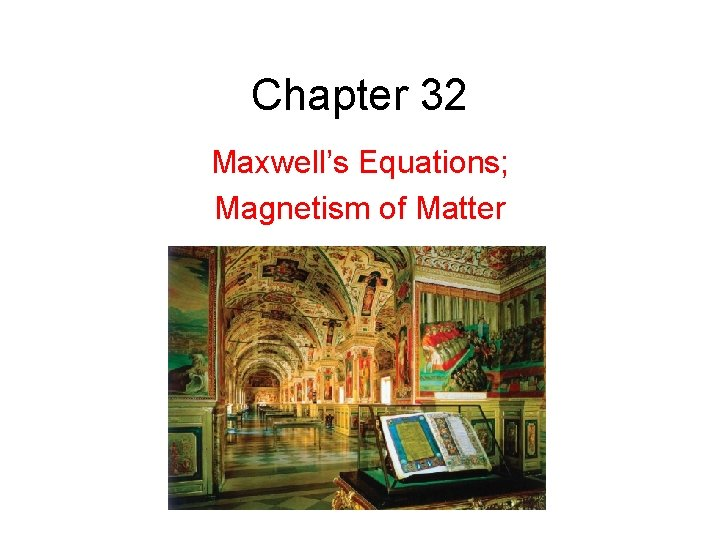Chapter 32 Maxwell’s Equations; Magnetism of Matter32. 2: Gauss’ Law for Magnetic Particles: The law asserts that the net magnetic flux FB through any closed Gaussian surface is zero. Here B is the magnetic field. Fig 32 -2 A bar magnet is a magnetic dipole. The iron filings suggest the magnetic field lines. (Colored light fills the background. ) (Richard Megna/Fundamental Photographs)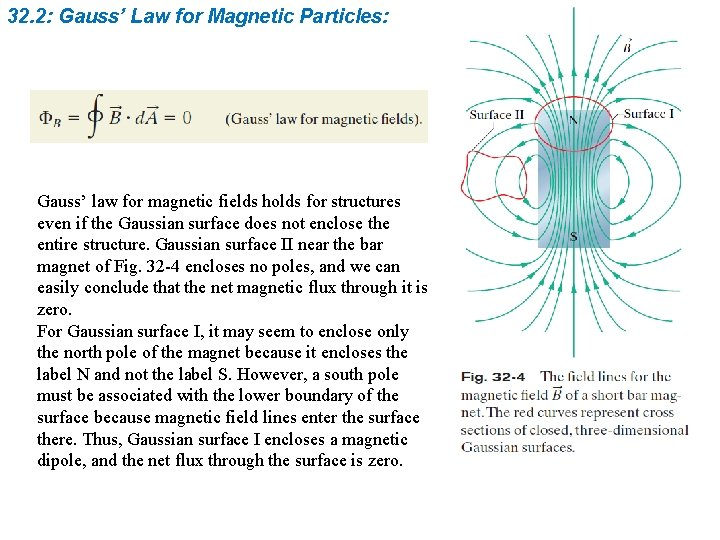32. 2: Gauss’ Law for Magnetic Particles: Gauss’ law for magnetic fields holds for structures even if the Gaussian surface does not enclose the entire structure. Gaussian surface II near the bar magnet of Fig. 32 -4 encloses no poles, and we can easily conclude that the net magnetic flux through it is zero. For Gaussian surface I, it may seem to enclose only the north pole of the magnet because it encloses the label N and not the label S. However, a south pole must be associated with the lower boundary of the surface because magnetic field lines enter the surface there. Thus, Gaussian surface I encloses a magnetic dipole, and the net flux through the surface is zero.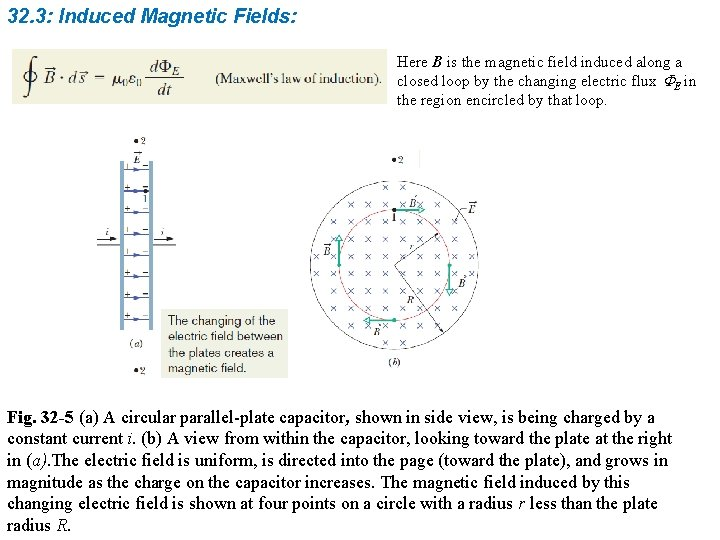32. 3: Induced Magnetic Fields: Here B is the magnetic field induced along a closed loop by the changing electric flux FE in the region encircled by that loop. Fig. 32 -5 (a) A circular parallel-plate capacitor, shown in side view, is being charged by a constant current i. (b) A view from within the capacitor, looking toward the plate at the right in (a). The electric field is uniform, is directed into the page (toward the plate), and grows in magnitude as the charge on the capacitor increases. The magnetic field induced by this changing electric field is shown at four points on a circle with a radius r less than the plate radius R.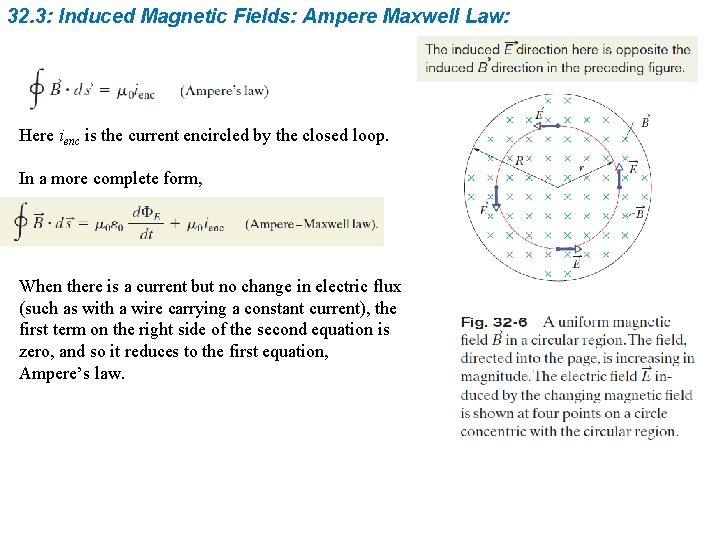32. 3: Induced Magnetic Fields: Ampere Maxwell Law: Here ienc is the current encircled by the closed loop. In a more complete form, When there is a current but no change in electric flux (such as with a wire carrying a constant current), the first term on the right side of the second equation is zero, and so it reduces to the first equation, Ampere’s law.32. 4: Displacement Current: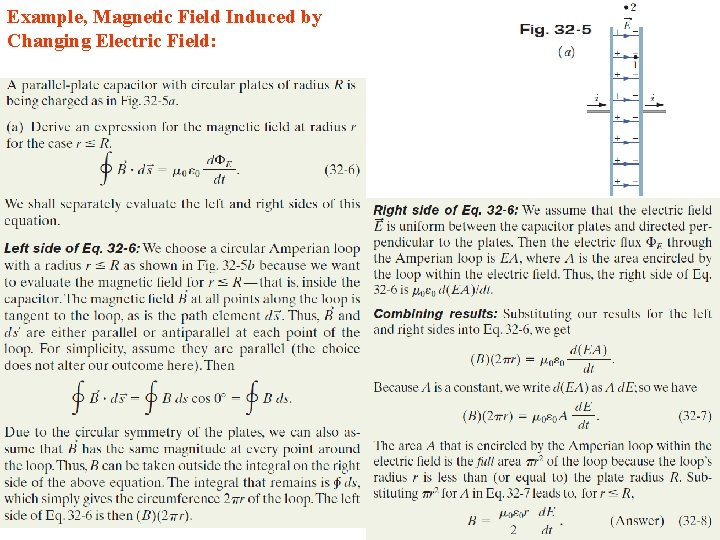Example, Magnetic Field Induced by Changing Electric Field: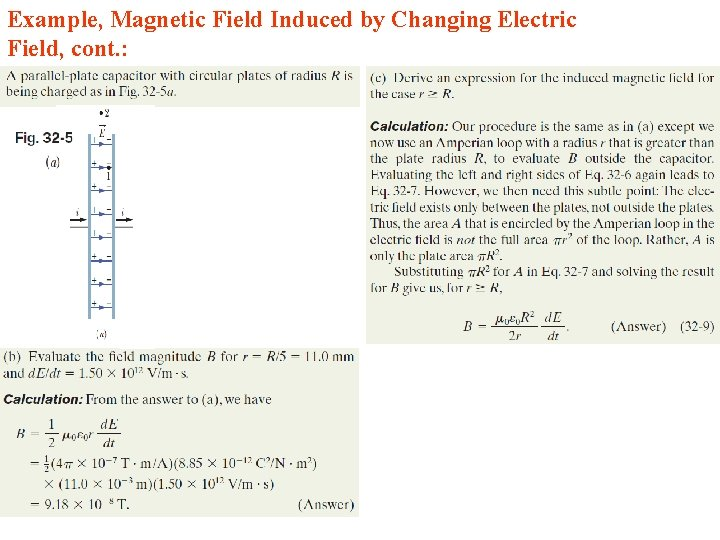Example, Magnetic Field Induced by Changing Electric Field, cont. :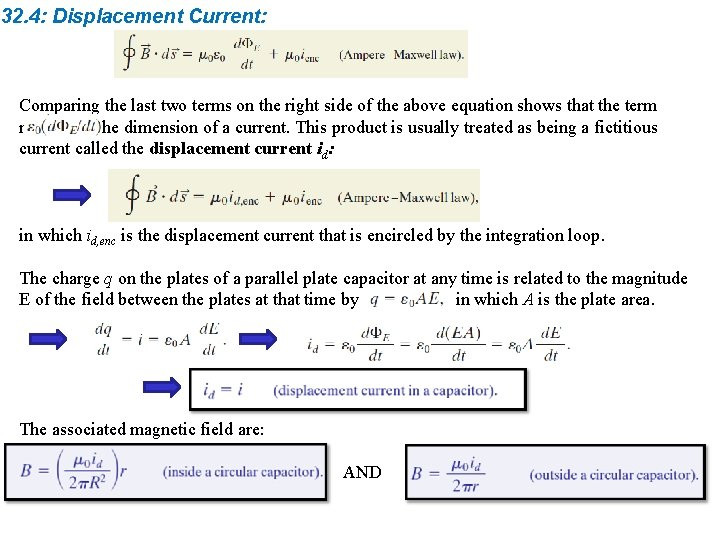32. 4: Displacement Current: Comparing the last two terms on the right side of the above equation shows that the term must have the dimension of a current. This product is usually treated as being a fictitious current called the displacement current id: in which id, enc is the displacement current that is encircled by the integration loop. The charge q on the plates of a parallel plate capacitor at any time is related to the magnitude E of the field between the plates at that time by in which A is the plate area. The associated magnetic field are: ANDExample, Treating a Changing Electric Field as a Displacement Current: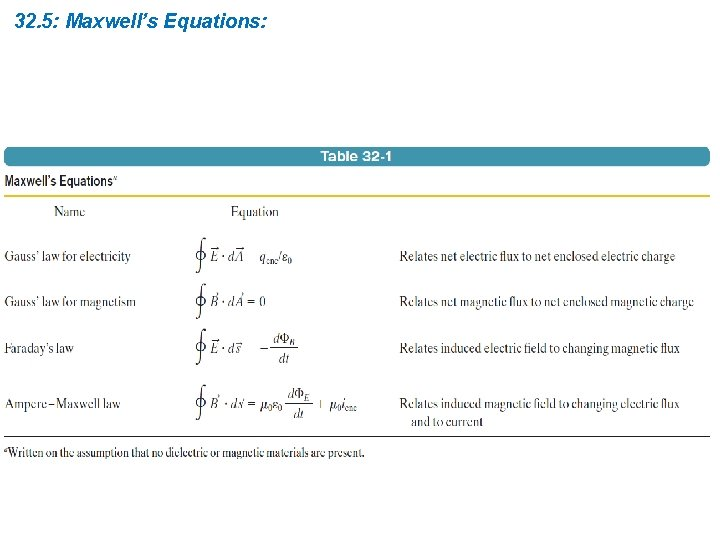32. 5: Maxwell’s Equations:32. 6: Magnets: The Magnetism of Earth: Because Earth’s magnetic field is that of a magnetic dipole, a magnetic dipole moment m is associated with the field. The field declination is the angle (left or right) between geographic north (which is toward 90° latitude) and the horizontal component of the field. The field inclination is the angle (up or down) between a horizontal plane and the field’s direction. Magnetometers measure these angles and determine the field with much precision. One can do reasonably well with just a compass and a dip meter. The point where the field is perpendicular to Earth’s surface and inward is not located at the geomagnetic north pole off Greenland as expected; instead, this socalled dip north pole is located in the Queen Elizabeth Islands in northern Canada, far from Greenland.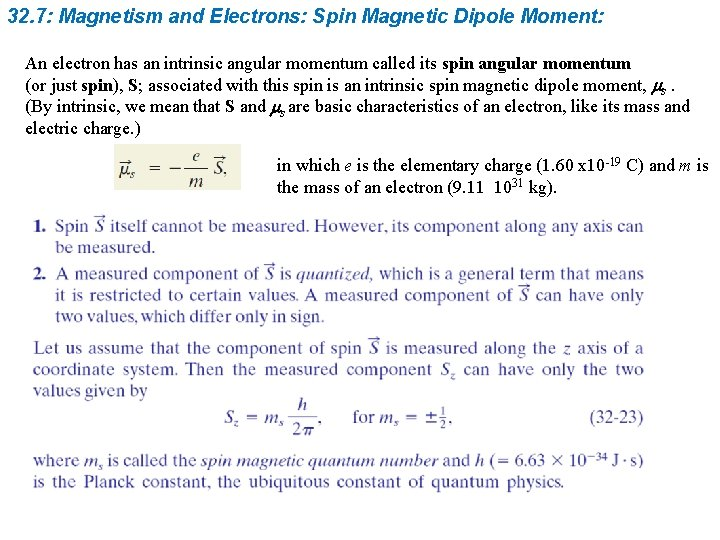32. 7: Magnetism and Electrons: Spin Magnetic Dipole Moment: An electron has an intrinsic angular momentum called its spin angular momentum (or just spin), S; associated with this spin is an intrinsic spin magnetic dipole moment, ms. (By intrinsic, we mean that S and ms are basic characteristics of an electron, like its mass and electric charge. ) in which e is the elementary charge (1. 60 x 10 -19 C) and m is the mass of an electron (9. 11 1031 kg).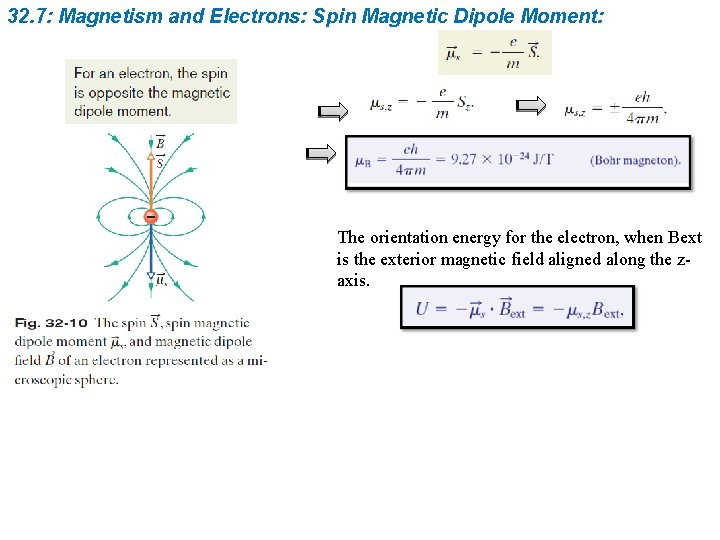32. 7: Magnetism and Electrons: Spin Magnetic Dipole Moment: The orientation energy for the electron, when Bext is the exterior magnetic field aligned along the zaxis.32. 7: Magnetism and Electrons: Orbital Magnetic Dipole Moment: When it is in an atom, an electron has an additional angular momentum called its orbital angular momentum, Lorb. Associated with it is an orbital magnetic dipole moment, morb ; the two are related by Only the component along any axis of the orbital angular momentum can be measured, and that component is quantized in which is ml called the orbital magnetic quantum number and “limit” refers to its largest allowed integer value. Similarly, only the component of the magnetic dipole moment of an electron along an axis can be measured, and that component is quantized. The orientation energy is: where the z axis is taken in the direction of Bext.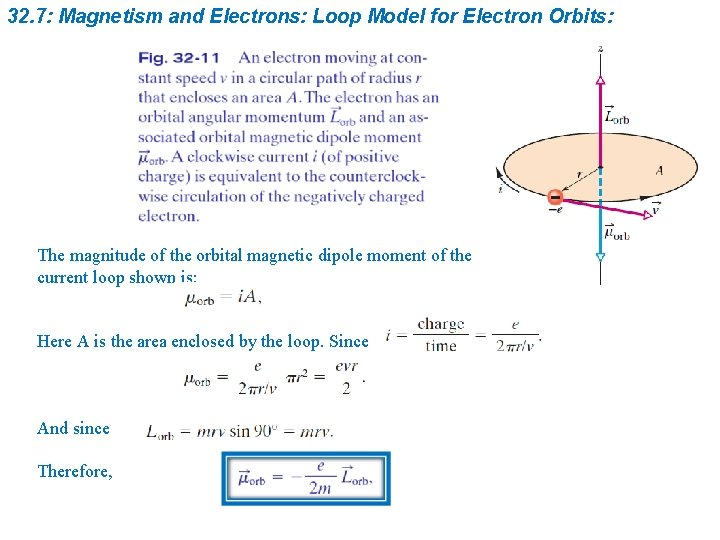32. 7: Magnetism and Electrons: Loop Model for Electron Orbits: The magnitude of the orbital magnetic dipole moment of the current loop shown is: Here A is the area enclosed by the loop. Since And since Therefore,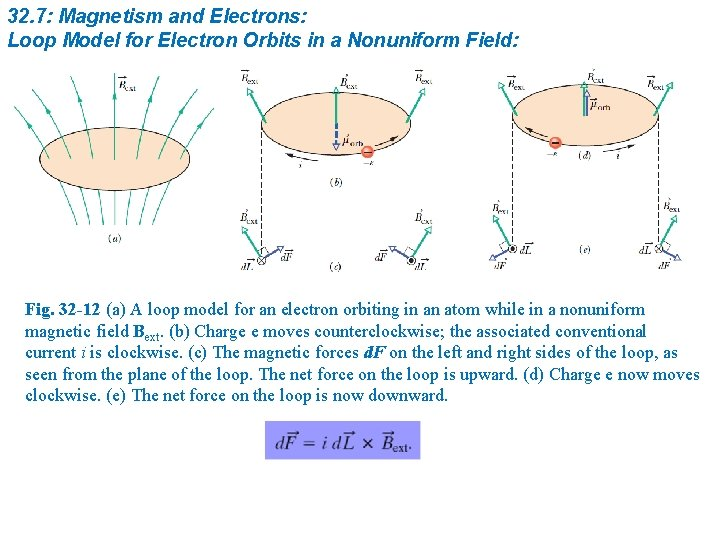32. 7: Magnetism and Electrons: Loop Model for Electron Orbits in a Nonuniform Field: Fig. 32 -12 (a) A loop model for an electron orbiting in an atom while in a nonuniform magnetic field Bext. (b) Charge e moves counterclockwise; the associated conventional current i is clockwise. (c) The magnetic forces d. F on the left and right sides of the loop, as seen from the plane of the loop. The net force on the loop is upward. (d) Charge e now moves clockwise. (e) The net force on the loop is now downward.32. 8: Magnetic Materials: Each electron in an atom has an orbital magnetic dipole moment and a spin magnetic dipole moment. The resultant of these two vectors combines with similar resultants for all other electrons in the atom, and the resultant for each atom combines with those for all the other atoms in a sample of a material. In a magnetic material the combination of all these magnetic dipole moments produces a magnetic field. There are three general types of magnetism. 1. Diamagnetism: In diamagnetism, weak magnetic dipole moments are produced in the atoms of the material when the material is placed in an external magnetic field Bext; the combination gives the material as a whole only a feeble net magnetic field. 2. Paramagnetism: Each atom of such a material has a permanent resultant magnetic dipole moment, but the moments are randomly oriented in the material and the material lacks a net magnetic field. An external magnetic field Bext can partially align the atomic magnetic dipole moments to give the material a net magnetic field. 3. Ferromagnetism: Some of the electrons in these materials have their resultant magnetic dipole moments aligned, which produces regions with strong magnetic dipole moments. An external field Bext can align the magnetic moments of such regions, producing a strong magnetic field for the material.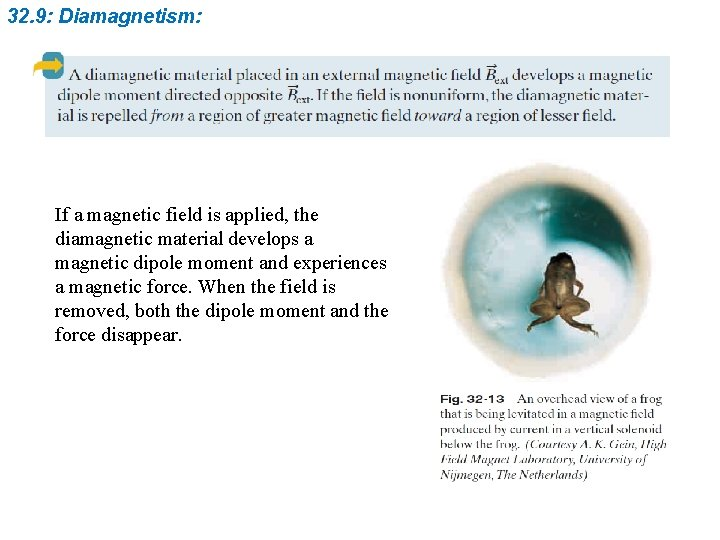32. 9: Diamagnetism: If a magnetic field is applied, the diamagnetic material develops a magnetic dipole moment and experiences a magnetic force. When the field is removed, both the dipole moment and the force disappear.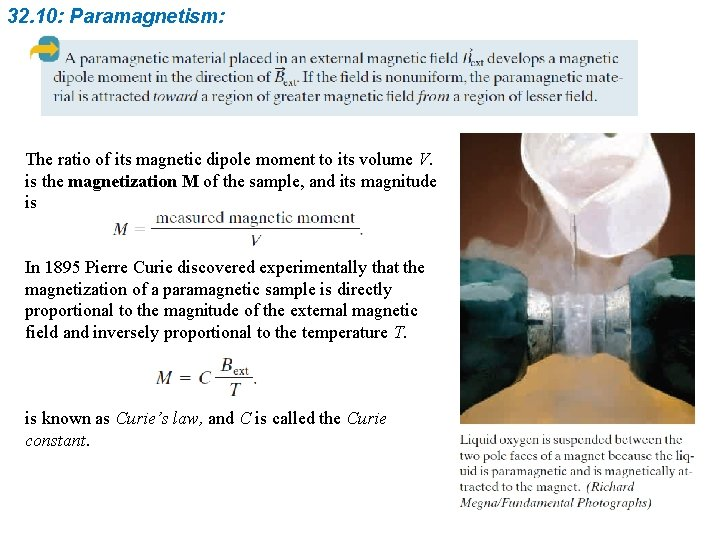32. 10: Paramagnetism: The ratio of its magnetic dipole moment to its volume V. is the magnetization M of the sample, and its magnitude is In 1895 Pierre Curie discovered experimentally that the magnetization of a paramagnetic sample is directly proportional to the magnitude of the external magnetic field and inversely proportional to the temperature T. is known as Curie’s law, and C is called the Curie constant.32. 10: Paramagnetism: Curie’s law is reasonable in that increasing Bext tends to align the atomic dipole moments in a sample and thus to increase M, whereas increasing T tends to disrupt the alignment via thermal agitation and thus to decrease M. However, the law is actually an approximation that is valid only when the ratio Bext/T is not too large.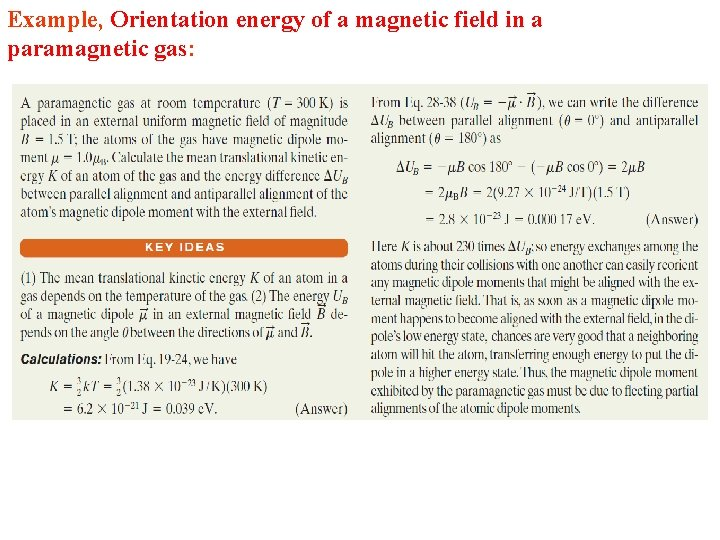Example, Orientation energy of a magnetic field in a paramagnetic gas:32. 11: Ferromagnetism: In ferromagnetic materials a quantum physical effect called exchange coupling is present where the electron spins of one atom interact with those of neighboring atoms. The result is alignment of the magnetic dipole moments of the atoms, in spite of the randomizing tendency of atomic collisions due to thermal agitation. This persistent alignment is what gives ferromagnetic materials their permanent magnetism. If the temperature of a ferromagnetic material is raised above a certain critical value, called the Curie temperature, the exchange coupling ceases to be effective. Most such materials then become simply paramagnetic.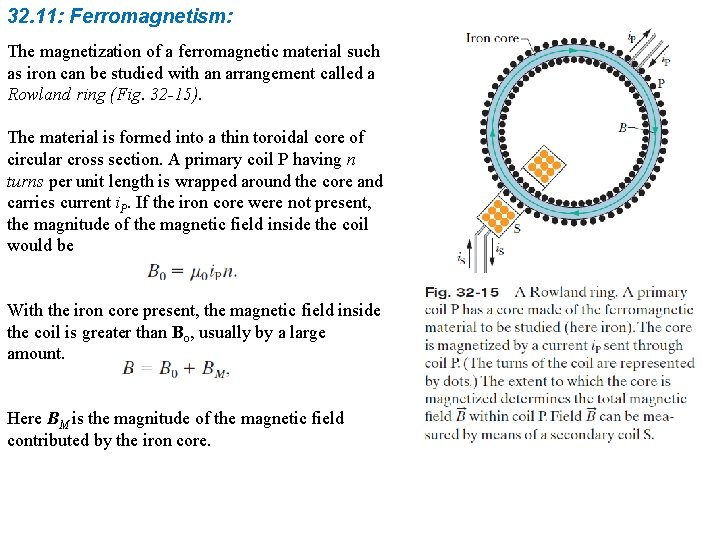32. 11: Ferromagnetism: The magnetization of a ferromagnetic material such as iron can be studied with an arrangement called a Rowland ring (Fig. 32 -15). The material is formed into a thin toroidal core of circular cross section. A primary coil P having n turns per unit length is wrapped around the core and carries current i. P. If the iron core were not present, the magnitude of the magnetic field inside the coil would be With the iron core present, the magnetic field inside the coil is greater than Bo, usually by a large amount. Here BM is the magnitude of the magnetic field contributed by the iron core.32. 11: Ferromagnetism: The ratio BM/BM, max, where BM, max is the maximum possible value of BM, corresponding to saturation, is plotted versus B 0. The curve is like the magnetization curve for a paramagnetic substance: Both curves show the extent to which an applied magnetic field can align the atomic dipole moments of a material. For the ferromagnetic core yielding Fig. 3216, the alignment of the dipole moments is about 70% complete for B 0 ≈ 1 x 10 -3 T. If B 0 were increased to 1 T, the alignment would be almost complete (but B 0 = 1 T, and thus almost complete saturation, is quite difficult to obtain).32. 11: Ferromagnetism: Magnetic Domains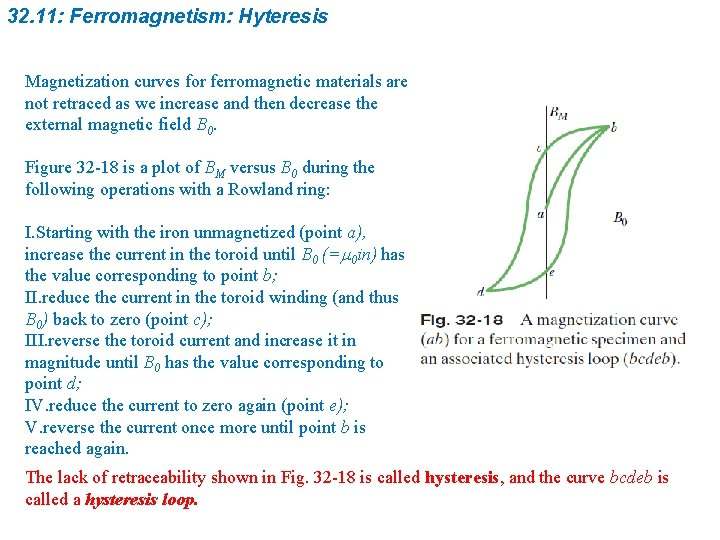32. 11: Ferromagnetism: Hyteresis Magnetization curves for ferromagnetic materials are not retraced as we increase and then decrease the external magnetic field B 0. Figure 32 -18 is a plot of BM versus B 0 during the following operations with a Rowland ring: I. Starting with the iron unmagnetized (point a), increase the current in the toroid until B 0 (=m 0 in) has the value corresponding to point b; II. reduce the current in the toroid winding (and thus B 0) back to zero (point c); III. reverse the toroid current and increase it in magnitude until B 0 has the value corresponding to point d; IV. reduce the current to zero again (point e); V. reverse the current once more until point b is reached again. The lack of retraceability shown in Fig. 32 -18 is called hysteresis, and the curve bcdeb is called a hysteresis loop.Example, Magnetic Dipole Moment in a Compass Needle: